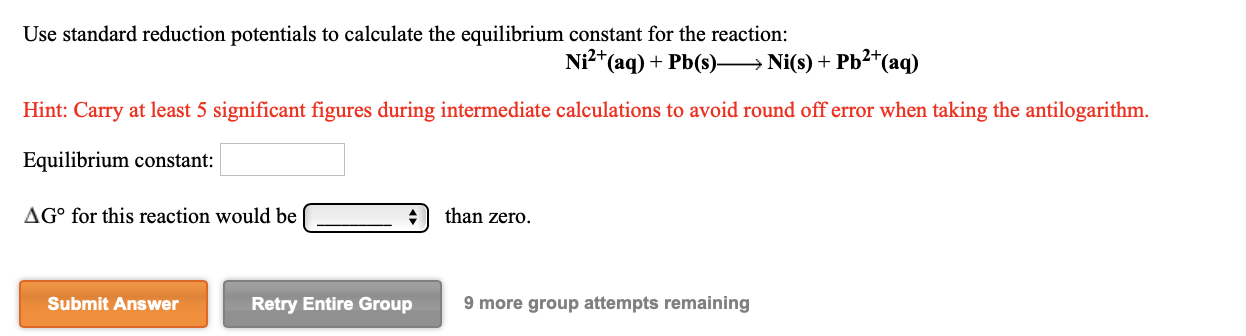# Use standard reduction potentials to calculate the equilibrium constant for the reaction: Ni²*(aq) + Pb(s)→ Ni(s) + Pb2*(aq) Hint: Carry at least 5 significant figures during intermediate calculations to avoid round off error when taking the antilogarithm. Equilibrium constant: AG° for this reaction would be than zero. Submit Answer Retry Entire Group 9 more group attempts remaining

Questionhelp_outlineImage TranscriptioncloseUse standard reduction potentials to calculate the equilibrium constant for the reaction: Ni²*(aq) + Pb(s)→ Ni(s) + Pb2*(aq) Hint: Carry at least 5 significant figures during intermediate calculations to avoid round off error when taking the antilogarithm. Equilibrium constant: AG° for this reaction would be than zero. Submit Answer Retry Entire Group 9 more group attempts remaining fullscreen

### Want to see this answer and more?

Experts are waiting 24/7 to provide step-by-step solutions in as fast as 30 minutes!*

*Response times vary by subject and question complexity. Median response time is 34 minutes and may be longer for new subjects.
Tagged in
ScienceChemistry

### Electrochemistry